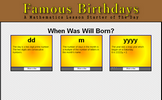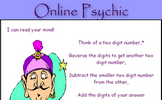# Measures of location

### Term 3 starting in week 11 :: Estimated time: 2 weeks

• Understand and use the mean, median and mode
• Choose the most appropriate average
• Identify outliers
• Compare distributions using averages and the range

For higher-attaining pupils:

• Find the mean from an ungrouped frequency table
• Find the mean from an grouped frequency table

This page should remember your ticks from one visit to the next for a period of time. It does this by using Local Storage so the information is saved only on the computer you are working on right now.

## Lesson Starters

Here are some suggestions for whole-class, projectable resources which can be used at the beginnings of each lesson in this block.

### 1st Lesson#### Average Smile

Calculate the mean, median and range of the given scores.

### 2nd Lesson#### Hot Summer Test

Write out a large times table. Get as far as possible in 5 minutes.

### 3rd Lesson#### Birthday Clues

Work out the date Will was born by answering some number questions.

### 4th Lesson#### Starting With

Write down as many mathematical words as possible that begin with a particular letter.

### 5th Lesson#### Mathster Mind

Guess the four digit number with help from the clues.

### 6th Lesson#### Mystic Maths

Work out why subtracting a two digit number from its reverse gives a multiple of nine.

Some of the Starters above are to reinforce concepts learnt, others are to introduce new ideas while others are on unrelated topics designed for retrieval practice or and opportunity to develop problem-solving skills.

White Rose ResourcesEnd of block assessments provide a quick progress check at the end of each block of learning to make sure students have understood the content covered. This Scheme of Learning was produced by White Rose Maths and is used here with permission granted on 30th June 2021.For All: